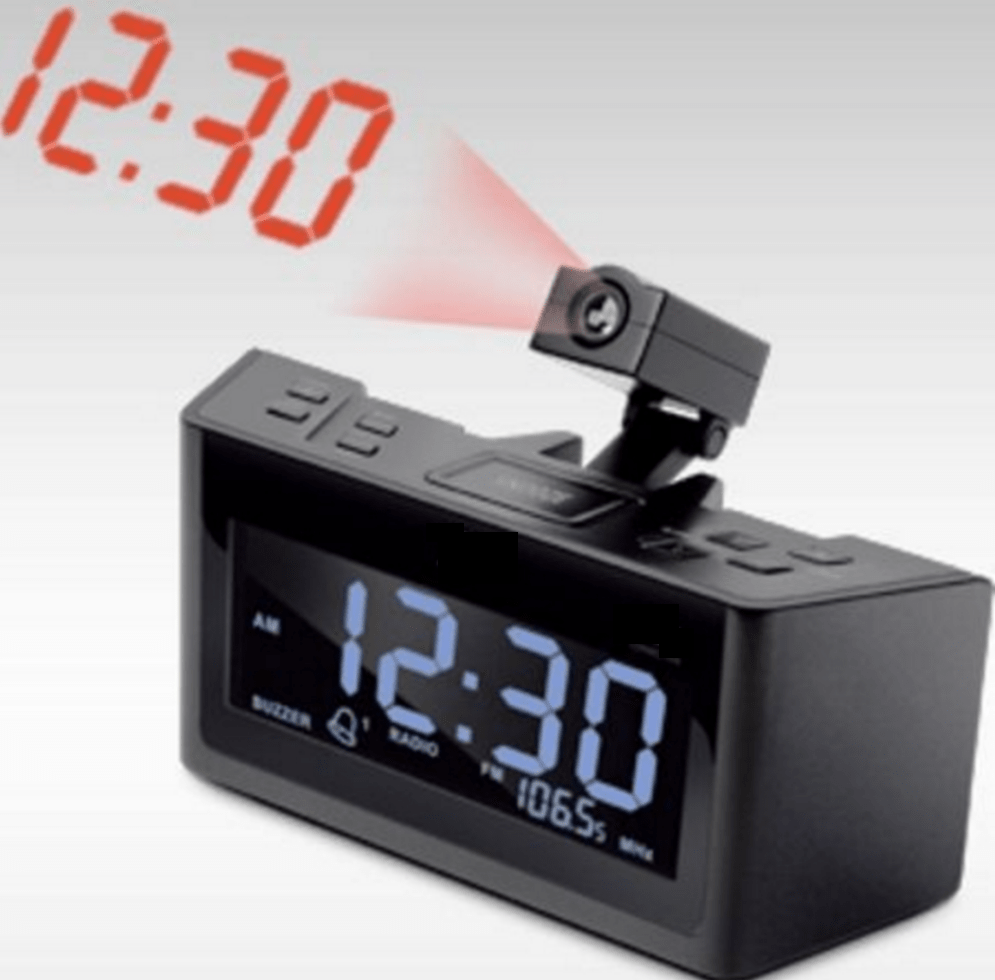# Digital clock mathemathics

If you’re like me, you definitely have such a digital clock somewhere in your house, most probably near your bed.Ever wondered what is the maximum number of segments of the clock that are used at a certain hour? And what time is it then?

I did… and here is how I count them.

I wrote a Perl script that calculates the number of segments of each hour and each minute: 24h x 60 min.

Each number has maximum 7 segments. This would be the number 8.

Here is the number of segments for each digit 0..9 : 6,2,5,5,4,5,6,3,7,6;

Important rules:

• The midnight time is the only exception when we have 00:xy
• the hour can be a single digit (1..9), but the minute will always be two digits. The minutes below 10 will be prefixed with 0.
• the 00:00 is the only time when we have 0X
• For example, 11 minutes after 1 AM is 1:11 and not 01:11

It turns out that the maximum amount of segments is 25 and the time is 8 minutes after midnight.

The second place is with 24 segments.

Max digits: 24 at 00:00
Max digits: 24 at 00:06
Max digits: 25 at 00:08
Final Max digits: 25 at 00:08

Minimum segments:

6 segments at 1:11

Note: this assumes that we use 24h numbering and not AM / PM numbering.

I will make the same calculation with AM/PM. But this is for another post.

If someone wants the Perl script, I can make it available on request.

## More details

Segments  – Time

24 at 00:00
Max digits: 24 at 00:00
20 at 00:01
23 at 00:02
23 at 00:03
22 at 00:04
23 at 00:05
24 at 00:06
Max digits: 24 at 00:06
21 at 00:07
25 at 00:08
Max digits: 25 at 00:08
24 at 00:09
20 at 00:10
16 at 00:11
19 at 00:12
19 at 00:13
18 at 00:14
19 at 00:15
20 at 00:16
17 at 00:17
21 at 00:18
20 at 00:19
23 at 00:20
19 at 00:21
22 at 00:22
22 at 00:23
21 at 00:24
22 at 00:25
23 at 00:26
20 at 00:27
24 at 00:28
23 at 00:29
23 at 00:30
19 at 00:31
22 at 00:32
22 at 00:33
21 at 00:34
22 at 00:35
23 at 00:36
20 at 00:37
24 at 00:38
23 at 00:39

19 at 23:43
18 at 23:44
19 at 23:45
20 at 23:46
17 at 23:47
21 at 23:48
20 at 23:49
21 at 23:50
17 at 23:51
20 at 23:52
20 at 23:53
19 at 23:54
20 at 23:55
21 at 23:56
18 at 23:57
22 at 23:58
21 at 23:59
Final Max digits: 25 at 00:08